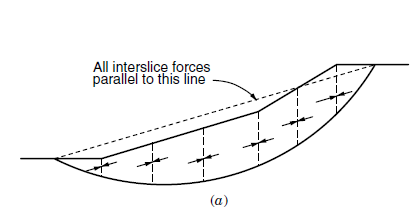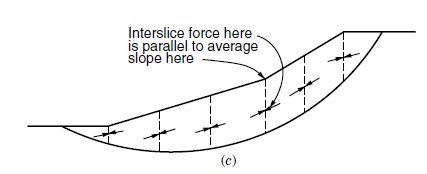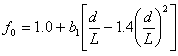Join one of our upcoming courses taking place around the world! Explore now

# Analysis Methods

The Methods page in the Project Settings dialog allows you to select the limit equilibrium analysis method(s) to be used for the Slide2 analysis. The following options are available.

• You may choose one or more Vertical Slice methods OR the Sarma Non-Vertical Slice method.
• Convergence options (number of slices, tolerance, maximum iterations)
• Interslice force function for GLE method
• Interslice strength function and slice angle options for Sarma method

Additional analysis options are available on the Advanced page of the Project Settings dialog.

## Vertical Slice Methods

The following vertical slice limit equilibrium analysis methods are available in Slide2:

• Ordinary/Fellenius
• Bishop Simplified
• Janbu Simplified
• Janbu Corrected
• Spencer
• Corps of Engineers #1
• Corps of Engineers #2
• Lowe-Karafiath
• GLE (General Limit Equilibrium) / Morgenstern-Price (the implementation of the GLE method in Slide2 is essentially equivalent to the Morgenstern-Price method)
• Sarma (Vertical Slice Method)

Any or all of these methods can be selected for a given Slide2 analysis. When Compute is run, all of the selected analysis methods will be simultaneously run on the model, and results for all methods will be available for viewing in the Slide2 Interpret program.

An excellent review of limit equilibrium analysis methods for slope stability can be found in the text by Abramson, Lee, Sharma & Boyce (2001). This includes the equations, theory and assumptions associated with each method.

Important note on computing multiple methods:

When computing multiple methods at once (e.g. Spencer, GLE, and Bishop), it's possible that the user may get different results when compared to computing a single method alone (e.g. Spencer only). The reason for this is that at the end of the computation, Slide2 takes all the global minima surfaces from all methods, and computes them with the other methods, in case one turns out to be a better surface.

For example, say at the end of a computation where Spencer, GLE, and Bishop were used, Spencer finds Surface 1 as the global minimum. GLE finds Surface 2. Bishop finds Surface 3.

Subsequently, Surfaces 2 and 3 are recomputed with Spencer. Surfaces 1 and 3 are recomputed with GLE. Surfaces 1 and 2 are recomputed with Bishop.

For Spencer, if Surface 2 gives a lower factor of safety than the original Surface 1, then Surface 2 will be displayed as the global minimum. On the other hand, if the user was computing Spencer alone, Surface 1 would be the global minimum. The same process applies to the other methods.

## Sarma Non-Vertical Slice Method

For a non-vertical slice analysis, you can choose the Sarma Non-Vertical Slice option. When this option is selected, the vertical slice methods will not be available (i.e. you cannot analyze vertical and non-vertical slices simultaneously on the same Slide2 model). For the Sarma Non-Vertical Slice method, you can also define Slice Angle options and Interslice Strength options.

## Corps of Engineers #1 and #2

The Corp. of Engineers #1 and #2 are also known as the Army Corp Modified Swedish Procedure. Basically, Corp of Engineers #1 is the assumption in the figure below:And Corp of Engineers #2 is the assumption in the figure below:These are legacy methods and are not recommended.

## Janbu Corrected Method

For a given slip surface, the Janbu Corrected safety factor is obtained by multiplying the Janbu Simplified safety factor for the surface, by a modification factor f0 , as follows:

Janbu Corrected Safety Factor = f0 *Janbu Simplified Safety Factor

The modification factor f0 is a function of the slope geometry, and the strength parameters of the soil, as illustrated below.

The Janbu modification factor is an attempt to compensate for the fact that the Janbu Simplified method satisfies only force equilibrium, and assumes zero interslice shear forces. Janbu developed a rigorous procedure, the Janbu Generalized method, satisfying both moment and force equilibrium. By comparing results using both the Janbu Simplified method and the Janbu Generalized method, for homogeneous slopes, Janbu was able to plot a series of curves (see below) described by the following equation:where the value of b1 varies according to the soil type as follows:

• c only soils: b1 = 0.69
• Φ only soils: b1 = 0.31
• c and Φ soils: b1 = 0.50
This derivation is based on calculations for a homogeneous (single material) slope. For multiple material slopes, where the slip surface passes through different soil types (for example, c only AND c - Φ soils), the relationship for c-Φ soils is used (b1 = 0.50)

## GLE Interslice Force Function

If the GLE analysis method is selected, you may specify the Interslice Force Function to be used in the analysis. By default, a Half Sine Interslice Force Function will be in effect, unless you specify a different function with the Change button. See the Interslice Force Function topic for details.

## Number of Slices

The Number of Slices is the number of vertical slices into which the sliding mass for each slip surface will be divided. The default number of 50 slices is sufficient to obtain an accurate solution for most problems. A large Number of Slices (e.g. greater than 100) is not recommended, since the accuracy of the solution will not necessarily be improved, and the computation time and size of the output file are directly proportional to the Number of Slices.

## Tolerance

The Tolerance is the difference in safety factor, between two successive iterations of the limit equilibrium analysis procedure, at which the solution is considered to have converged, and the iteration process is stopped. The default value of 0.005 is recommended. Much smaller values will increase the computation time and may lead to convergence problems. Values greater than 0.01 will speed up the computation but will lead to less precise values of the safety factor.

## Maximum Number of Iterations

The Maximum Iterations is the maximum number of iterations allowed in the limit equilibrium analysis, for each slip surface. Although the default value is 75, it should be noted that for a typical problem, only 3 to 4 iterations are required for convergence. If a given slip surface requires more than say, 20 iterations, incorrect material properties, or very low safety factors, may be the cause, the user should check their model input carefully. If the Maximum Number of Iterations is reached, then the iteration process is terminated for that slip surface, and the last calculated value of the safety factor is recorded.